## (10) 朴素贝叶斯

news/2023/12/1 12:30:29

### 文章目录

• 1 概述
• 2 不同分布下的贝叶斯
• 2.1 高斯朴素贝叶斯GaussianNB
• 2.1.1 认识高斯朴素贝叶斯
• 2.1.2 探索贝叶斯：高斯朴素贝叶斯擅长的数据集
• 2.1.3 探索贝叶斯：高斯朴素贝叶斯的拟合效果与运算速度
• 2.2 概率类模型的评估指标
• 2.2.1 布里尔分数Brier Score
• 2.2.2 对数损失Log Loss
• 2.2.3 可靠性曲线Reliability Curve
• 2.2.4 预测概率的直方图
• 2.2.5 校准可靠性曲线
• 2.3 多项式朴素贝叶斯以及其变化
• 2.3.1 多项式朴素贝叶斯MultinomialNB
• 2.3.2 伯努利朴素贝叶斯BernoulliNB
• 2.3.3 探索贝叶斯：样本不均衡问题
• 2.3.4 改进多项式朴素贝叶斯：补集朴素贝叶斯ComplementNB
• 3 案例：贝叶斯分类器做文本分类
• 3.1 文本编码技术简介
• 3.1.1 单词计数向量
• 3.1.2 TF-IDF
• 3.2 探索文本数据
• 3.3 使用TF-IDF将文本数据编码
• 3.4 在贝叶斯上分别建模，查看结果

# 1 概述

1. 贝叶斯定理： 朴素贝叶斯算法基于贝叶斯定理，其中通过观察到的特征预测未观察到的类别。贝叶斯定理表达式如下：
P ( y ∣ X ) = P ( X ∣ y ) ⋅ P ( y ) P ( X ) P(y|X) = \frac{{P(X|y) \cdot P(y)}}{{P(X)}}
其中，P(y|X) 是给定特征 X 条件下类别 y 的概率，P(X|y) 是类别 y 条件下特征 X 的概率，P(y) 是类别 y 的先验概率，P(X) 是特征 X 的边缘概率。

2. 特征条件独立性假设： 朴素贝叶斯算法假设给定类别的特征之间是条件独立的，即每个特征对于类别的影响是相互独立的。这种假设简化了模型的计算，并降低了数据要求。

3. 模型训练： 在朴素贝叶斯算法中，首先需要从带有标签的训练数据中学习类别的先验概率和特征的条件概率。根据训练数据，计算每个类别的先验概率 P(y) 和每个特征在给定类别下的条件概率 P(X|y)。

4. 特征表示： 在使用朴素贝叶斯算法时，需要将输入数据表示为特征向量。常用的表示方式是使用词袋模型（Bag-of-Words），其中每个特征对应一个词语或词语的存在与否。

5. 预测： 在进行预测时，朴素贝叶斯算法根据贝叶斯定理和特征条件独立性假设计算后验概率 P(y|X)，并选择具有最高后验概率的类别作为预测结果。

• 算法简单且易于实现。
• 对于小规模数据集表现良好，适用于处理高维数据。
• 具有良好的可解释性。

• 特征条件独立性假设可能不符合实际情况，因此在某些数据集上性能可能较差。
• 对于特征之间存在依赖关系的问题，朴素贝叶斯算法无法准确建模。
• 对于稀疏数据和缺失数据的处理相对较差。

# 2 不同分布下的贝叶斯

## 2.1 高斯朴素贝叶斯GaussianNB

### 2.1.1 认识高斯朴素贝叶斯

class sklearn.naive_bayes.GaussianNB (priors=None, var_smoothing=1e-09)

P ( x i ∣ Y ) = 1 2 π σ y 2 e x p ( − ( x i − μ y ) 2 2 σ y 2 ) P(x_i|Y) = \frac{1}{\sqrt{2\pi\sigma^2_{y}}}exp(-\frac{(x_i-\mu_{y})^2}{2\sigma^2_{y}})

• priors：先验概率，如果不输入，则根据训练数据得到先验概率
• var_smoothing：浮点数，可不填（默认值=1e-9）在估计方差时，为了追求估计的稳定性，将所有特征的方差中最大的方差以某个比例添加到估计的方差中。这个比例，由var_smoothing参数控制。

• 导入需要的库和数据
from sklearn.naive_bayes import GaussianNB
from sklearn.model_selection import train_test_splitdigits = load_digits()
X, y = digits.data, digits.targetX_train, X_test, y_train, y_test = train_test_split(X, y, random_state=420)

• 建模，探索建模结果
gnb = GaussianNB().fit(X_train, y_train)# 查看预测准确率
acc_score = gnb.score(X_test, y_test)
print("预测得分为：", acc_score)
# 查看预测结果
y_pred = gnb.predict(X_test)
print("预测结果为：", y_pred[:20])
# 查看预测的概率结果
prob = gnb.predict_proba(X_test)  # 每一列对应一个标签的概率，每一行的概率加和都为1
print('预测各类别下的概率：', prob.shape)

预测得分为： 0.8511111111111112


• 使用混淆矩阵来查看贝叶斯的分类结果
from sklearn.metrics import confusion_matrix as CMprint(CM(y_test, y_pred))  # 对角线上的数字表示预测正确的数量，非对角线上的数字表示预测错误的数量# 注意，ROC曲线是不能用于多分类的。多分类状况下最佳的模型评估指标是混淆矩阵和整体的准确率。

[[40  0  0  0  0  0  0  1  0  0][ 0 40  2  0  0  0  0  0  3  2][ 0  3 27  1  0  0  1  0 14  0][ 0  0  0 31  0  1  0  2  3  0][ 0  0  1  0 33  0  1  4  0  0][ 0  0  0  1  0 46  1  1  0  0][ 0  0  1  0  0  0 42  0  0  0][ 0  0  0  0  0  0  0 44  0  0][ 0  6  0  1  0  0  0  2 46  0][ 0  1  0  2  2  0  0  3  7 34]]


### 2.1.2 探索贝叶斯：高斯朴素贝叶斯擅长的数据集

import numpy as np
import matplotlib.pyplot as plt
from matplotlib.colors import ListedColormap
from sklearn.model_selection import train_test_split
from sklearn.preprocessing import StandardScaler
from sklearn.datasets import make_moons, make_circles, make_classification
from sklearn.naive_bayes import GaussianNB, MultinomialNB, BernoulliNB, ComplementNBh = .02
names = ["Multinomial", "Gaussian", "Bernoulli", "Complement"]
classifiers = [MultinomialNB(), GaussianNB(), BernoulliNB(), ComplementNB()]
X, y = make_classification(n_features=2, n_redundant=0, n_informative=2, random_state=1, n_clusters_per_class=1)
rng = np.random.RandomState(2)
X += 2 * rng.uniform(size=X.shape)  # 加点噪声
linearly_separable = (X, y)  # 合并成元组
datasets = [make_moons(noise=0.3, random_state=0), make_circles(noise=0.2, factor=0.5, random_state=1),linearly_separable]figure = plt.figure(figsize=(6, 9))
i = 1for ds_index, ds in enumerate(datasets):X, y = dsX = StandardScaler().fit_transform(X)  # 标准化X_train, X_test, y_train, y_test = train_test_split(X, y, test_size=.4, random_state=42)x1_min, x1_max = X[:, 0].min() - .5, X[:, 0].max() + .5x2_min, x2_max = X[:, 1].min() - .5, X[:, 1].max() + .5array1, array2 = np.meshgrid(np.arange(x1_min, x1_max, 0.2), np.arange(x2_min, x2_max, 0.2))cm = plt.cm.RdBucm_bright = ListedColormap(['#FF0000', '#0000FF'])ax = plt.subplot(len(datasets), 2, i)if ds_index == 0:ax.set_title("Input data")ax.scatter(X_train[:, 0], X_train[:, 1], c=y_train, cmap=cm_bright, edgecolors='k')ax.scatter(X_test[:, 0], X_test[:, 1], c=y_test, cmap=cm_bright, alpha=0.6, edgecolors='k')ax.set_xlim(array1.min(), array1.max())ax.set_ylim(array2.min(), array2.max())ax.set_xticks(())  # 去掉坐标轴ax.set_yticks(())i += 1ax = plt.subplot(len(datasets), 2, i)clf = GaussianNB().fit(X_train, y_train)score = clf.score(X_test, y_test)Z = clf.predict_proba(np.c_[array1.ravel(), array2.ravel()])[:, 1]Z = Z.reshape(array1.shape)ax.contourf(array1, array2, Z, cmap=cm, alpha=.8)ax.scatter(X_train[:, 0], X_train[:, 1], c=y_train, cmap=cm_bright, edgecolors='k')ax.scatter(X_test[:, 0], X_test[:, 1], c=y_test, cmap=cm_bright, edgecolors='k', alpha=0.6)ax.set_xlim(array1.min(), array1.max())ax.set_ylim(array2.min(), array2.max())ax.set_xticks(())ax.set_yticks(())if ds_index == 0:ax.set_title("Gaussian Bayes")ax.text(array1.max() - .3, array2.min() + .3, ('{:.1f}%'.format(score * 100)),size=15, horizontalalignment='right')i += 1plt.tight_layout()
plt.show()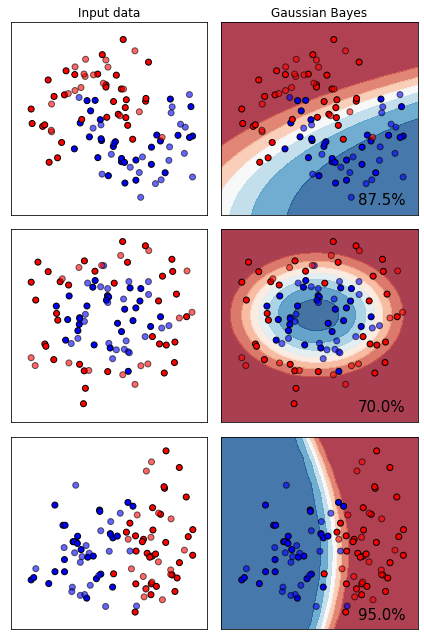从图上来看，高斯贝叶斯属于比较特殊的一类分类器，其分类效果在二分数据和月亮型数据上表现优秀，但是环形数据不太擅长。许多线性模型比如逻辑回归，线性SVM等等，在线性数据集上会绘制直线决策边界，因此难以对月亮型和环形数据进行区分，但高斯朴素贝叶斯的决策边界是曲线，可以是环形也可以是弧线，所以尽管贝叶斯本身更加擅长线性可分的二分数据，但朴素贝叶斯在环形数据和月亮型数据上也可以有远远胜过其他线性模型的表现。

### 2.1.3 探索贝叶斯：高斯朴素贝叶斯的拟合效果与运算速度

1. 首先导入需要的模块和库
import numpy as np
import matplotlib.pyplot as plt
from sklearn.naive_bayes import GaussianNB
from sklearn.svm import SVC
from sklearn.ensemble import RandomForestClassifier as RFC
from sklearn.tree import DecisionTreeClassifier as DTC
from sklearn.linear_model import LogisticRegression as LR
from sklearn.model_selection import learning_curve  # 画学习曲线的模块
from sklearn.model_selection import ShuffleSplit  # 设定交叉验证模式的模块
from time import time
import datetime

1. 定义绘制学习曲线的函数
# 输入分类器、数据、标题、横坐标、纵坐标、交叉验证、线程，一次性画出多个分类器的对比
def plot_learning_curve(estimator, title, X, y, ax,ylim=None,  #设置纵坐标的取值范围cv=None,  #交叉验证n_jobs=None  #设定索要使用的线程):train_sizes, train_scores, test_scores = learning_curve(estimator, X, y, cv=cv, n_jobs=n_jobs)# train_sizes:(5,)返回训练样本数，train_scores:(5,50)返回训练集上的分数，test_scores:(5,50)返回测试集上的分数ax.set_title(title)if ylim is not None:ax.set_ylim(*ylim)ax.set_xlabel("Training examples")ax.set_ylabel("Score")ax.grid()  #显示网格作为背景，不是必须ax.plot(train_sizes, np.mean(train_scores, axis=1), 'o-', color="r", label="Training score")ax.plot(train_sizes, np.mean(test_scores, axis=1), 'o-', color="g", label="Test score")ax.legend(loc="best")return ax

1. 导入数据，定义循环
digits = load_digits()
X, y = digits.data, digits.targettitle = ["Naive Bayes", "DecisionTree", "SVM, RBF kernel", "RandomForest", "Logistic"]
model = [GaussianNB(), DTC(), SVC(gamma=0.001), RFC(n_estimators=50), LR(C=.1, solver="lbfgs")]
cv = ShuffleSplit(n_splits=50, test_size=.2, random_state=0)

1. 进入循环，绘制学习曲线
fig, axes = plt.subplots(1, 5, figsize=(30, 6))
for ind, title_, estimator in zip(range(len(title)), title, model):times = time()plot_learning_curve(estimator, title_, X, y, ax=axes[ind], ylim=[0.7, 1.05], n_jobs=4, cv=cv)print("{}:{}".format(title_, datetime.datetime.fromtimestamp(time() - times).strftime("%M:%S:%f")))
plt.show()

Naive Bayes:00:01:365892
DecisionTree:00:01:478388
SVM, RBF kernel:00:08:597016
RandomForest:00:07:524288
Logistic:00:16:483629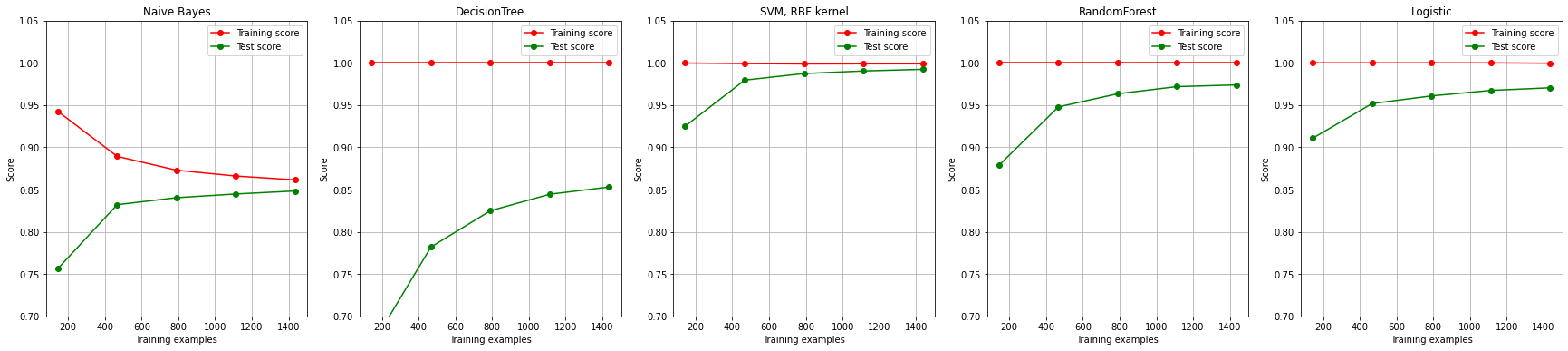可以看出：

## 2.2 概率类模型的评估指标

### 2.2.1 布里尔分数Brier Score

B r i e r S c o r e = 1 N ∑ t = 1 n ( p i − o i ) 2 Brier Score=\frac{1}{N}\sum_{t=1}^{n}(p_{i}-o_{i})^{2}

from sklearn.metrics import brier_score_loss  # 注意：只对二分类有效# 第一个参数是真实标签，第二个参数是预测的概率
# 在二分类情况下，接口predict_proba会返回两列，但SVC的接口decision_function却只会返回一列
# 要随时注意，使用了怎样的概率分类器，以辨别查找置信度的接口，以及这些接口的结构
brier_score_loss(y_test, prob[:, 1], pos_label=1)
# pos_label与prob中的索引一致，就可以查看这个类别下的布里尔分数是多少


from sklearn.svm import SVC
from sklearn.linear_model import LogisticRegression as LRlogi = LR(C=1., solver='lbfgs', max_iter=3000, multi_class='auto').fit(X_train, y_train)
svc = SVC(kernel="linear", gamma=1).fit(X_train, y_train)print(brier_score_loss(y_test, logi.predict_proba(X_test)[:, 1], pos_label=1))# 由于SVC的置信度并不是概率，为了可比性，我们需要将SVC置信度“距离”归一化，压缩到0到1之间
svc_prob = (svc.decision_function(X_test) - svc.decision_function(X_test).min()) / (svc.decision_function(X_test).max() - svc.decision_function(X_test).min())print(brier_score_loss(y_test, svc_prob[:, 1], pos_label=1))

ValueError: Only binary classification is supported. The type of the target is multiclass.


import pandas as pdname = ["Bayes", "Logistic", "SVC"]
color = ["red", "black", "orange"]
df = pd.DataFrame(index=range(10), columns=name)
for i in range(10):df.loc[i, name] = brier_score_loss(y_test, prob[:, i], pos_label=i)df.loc[i, name] = brier_score_loss(y_test, logi.predict_proba(X_test)[:, i], pos_label=i)df.loc[i, name] = brier_score_loss(y_test, svc_prob[:, i], pos_label=i)
for i in range(df.shape):plt.plot(range(10), df.iloc[:, i], c=color[i])
plt.legend()
plt.show()


### 2.2.2 对数损失Log Loss

− l o g P ( y t r u e ∣ y p r e d ) = − ( y t r u e ∗ l o g ( y p r e d ) + ( 1 − y t r u e ) ∗ l o g ( 1 − y p r e d ) ) -logP(y_{true}|y_{pred})=-(y_{true}*log(y_{pred})+(1-y_{true})*log(1-y_{pred}))

J ( θ ) = − ∑ i = 1 m ( y i ∗ l o g ( y θ ( x i ) ) + ( 1 − y i ) ∗ l o g ( 1 − y θ ( x i ) ) ) J(\theta)=-\sum_{i=1}^m(y_{i}*log(y_{\theta}(x_i))+(1-y_{i})*log(1-y_{\theta}(x_i)))

from sklearn.metrics import log_losslogi = LR(C=1., solver='lbfgs', max_iter=3000, multi_class='auto').fit(X_train, y_train)
svc = SVC(kernel="linear", gamma=1).fit(X_train, y_train)
svc_prob = (svc.decision_function(X_test) - svc.decision_function(X_test).min()) / (svc.decision_function(X_test).max() - svc.decision_function(X_test).min())log_loss(y_test, prob), log_loss(y_test, logi.predict_proba(X_test)), log_loss(y_test, svc_prob)

(2.364795042985354, 0.14190192654122152, 1.6078544571320117)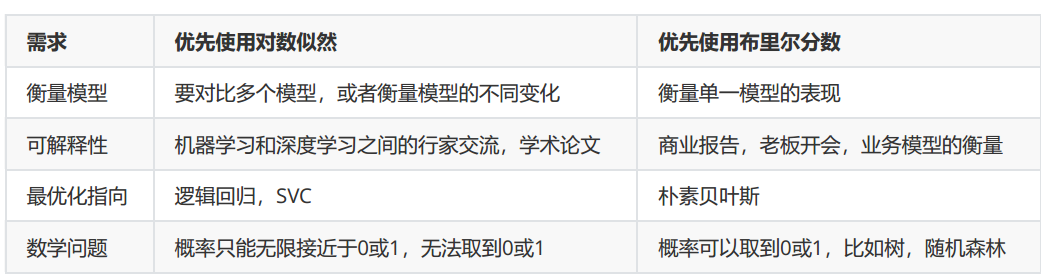### 2.2.3 可靠性曲线Reliability Curve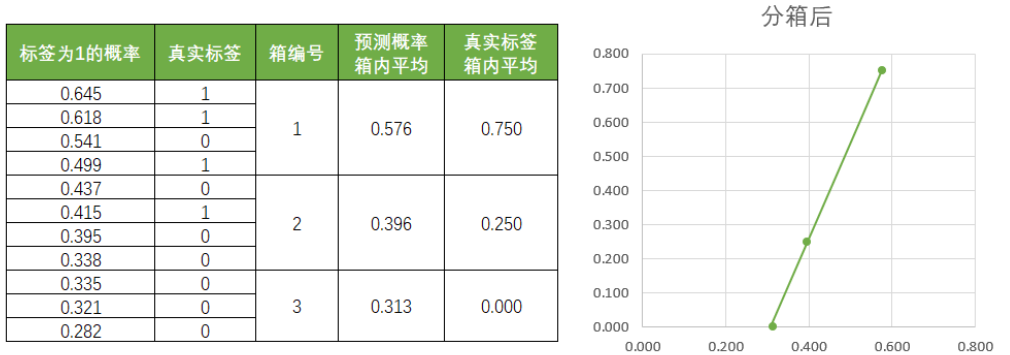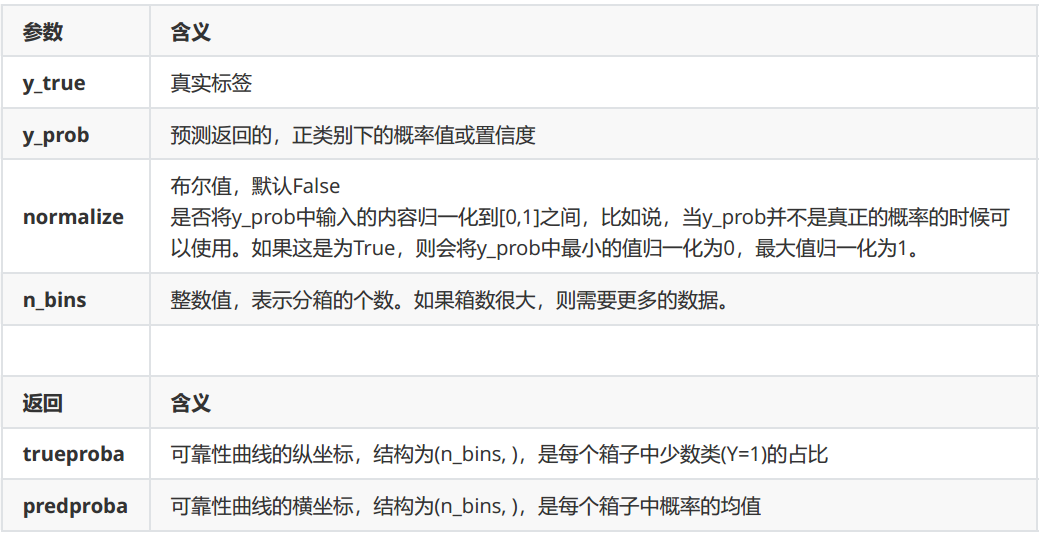import numpy as np
import matplotlib.pyplot as plt
from sklearn.datasets import make_classification as mc
from sklearn.naive_bayes import GaussianNB
from sklearn.model_selection import train_test_split
from sklearn.calibration import calibration_curve# 生成数据集
X, y = mc(n_samples=100000, n_features=20, n_classes=2, n_informative=2, n_redundant=10, random_state=42)# 样本量足够大，因此可以直接使用1%的样本作为训练集
X_train, X_test, y_train, y_test = train_test_split(X, y, test_size=0.99, random_state=42)gbn = GaussianNB().fit(X_train, y_train)
y_pred = gbn.predict(X_test)
prob_pos = gbn.predict_proba(X_test)[:, 1]  # 预测为1的概率# 从类calibration_curve中获取横纵坐标
prob_true, prob_pred = calibration_curve(y_test, prob_pos, n_bins=10)# 绘制图像
plt.figure()
ax1 = plt.subplot()
ax1.plot([0, 1], [0, 1], "k:", label="Perfectly calibrated")  # 绘制对角线,k:表示黑色虚线
ax1.plot(prob_pred, prob_true, "s-", label=f'Bayes ({round(gbn.score(X_test, y_test), 3)})')  # 绘制可靠性曲线
ax1.set_ylabel("True probability for class 1")
ax1.set_xlabel("Mean predicted probability")
ax1.set_ylim([-0.05, 1.05])
ax1.legend()
plt.show()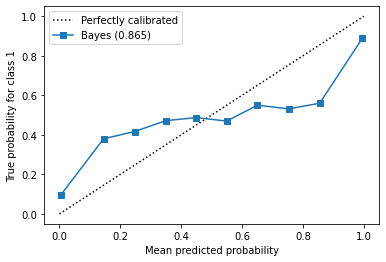不同的n_bins取值下曲线如何改变？

fig, axes = plt.subplots(1, 3, figsize=(18, 4))
for ind, i in enumerate([3, 10, 100]):prob_true, prob_pred = calibration_curve(y_test, prob_pos, n_bins=i)axes[ind].plot([0, 1], [0, 1], "k:", label="Perfectly calibrated")axes[ind].plot(prob_pred, prob_true, "s-", label=f'n_bins={i}')axes[ind].set_ylabel("True probability for class 1")axes[ind].set_xlabel("Mean predicted probability")axes[ind].set_ylim([-0.05, 1.05])axes[ind].legend()
plt.show()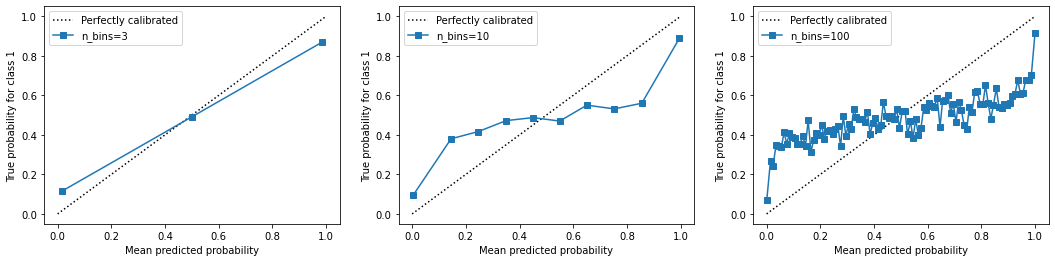from sklearn.svm import SVC
from sklearn.linear_model import LogisticRegression as LRname = ["GaussianBayes", "Logistic", "SVC"]
gnb = GaussianNB()
logi = LR(C=1., solver='lbfgs', max_iter=3000, multi_class="auto")
svc = SVC(kernel="linear", gamma=1)fig, ax1 = plt.subplots(figsize=(8, 6))
ax1.plot([0, 1], [0, 1], "k:", label="Perfectly calibrated")for clf, name_ in zip([gnb, logi, svc], name):clf.fit(X_train, y_train)y_pred = clf.predict(X_test)#hasattr(obj,name)：查看一个类obj中是否存在名字为name的接口，存在则返回Trueif hasattr(clf, "predict_proba"):prob_pos = clf.predict_proba(X_test)[:, 1]else:  # use decision functionprob_pos = clf.decision_function(X_test)prob_pos = (prob_pos - prob_pos.min()) / (prob_pos.max() - prob_pos.min())#返回布里尔分数clf_score = brier_score_loss(y_test, prob_pos, pos_label=1)trueproba, predproba = calibration_curve(y_test, prob_pos, n_bins=10)ax1.plot(predproba, trueproba, "s-", label="%s (%1.3f)" % (name_, clf_score))ax1.set_ylabel("True probability for class 1")
ax1.set_xlabel("Mean predcited probability")
ax1.set_ylim([-0.05, 1.05])
ax1.legend()
ax1.set_title('Calibration plots  (reliability curve)')
plt.show()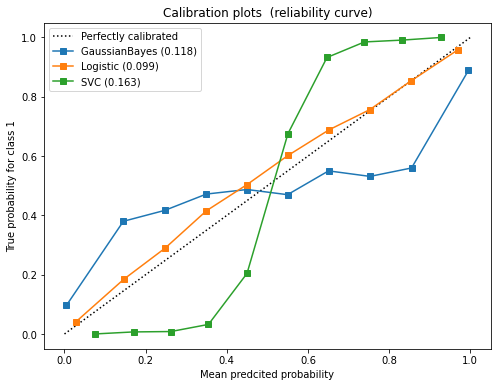### 2.2.4 预测概率的直方图

fig, ax2 = plt.subplots(figsize=(8, 6))for clf, name_ in zip([gnb, logi, svc], name):clf.fit(X_train, y_train)y_pred = clf.predict(X_test)#hasattr(obj,name)：查看一个类obj中是否存在名字为name的接口，存在则返回Trueif hasattr(clf, "predict_proba"):prob_pos = clf.predict_proba(X_test)[:, 1]else:  # use decision functionprob_pos = clf.decision_function(X_test)prob_pos = (prob_pos - prob_pos.min()) / (prob_pos.max() - prob_pos.min())ax2.hist(prob_pos  #预测概率, bins=10, label=name_, histtype="step"  #设置直方图为透明，防止直方图之间的覆盖, lw=2  #设置直方图每个柱子描边的粗细)ax2.set_ylabel("Distribution of probability")
ax2.set_xlabel("Mean predicted probability")
ax2.set_xlim([-0.05, 1.05])
ax2.set_xticks([0, 0.1, 0.2, 0.3, 0.4, 0.5, 0.6, 0.7, 0.8, 0.9, 1])
ax2.legend(loc=9)
plt.show()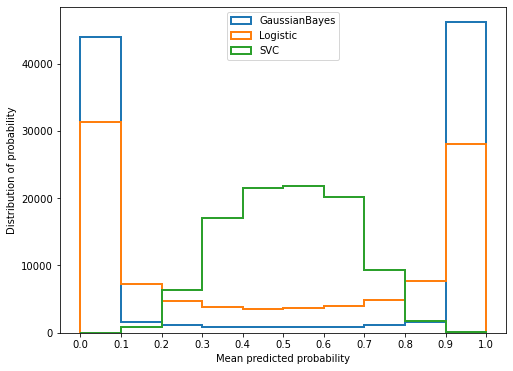### 2.2.5 校准可靠性曲线

sklearn.calibration.CalibratedClassifierCV(base_estimator=None,method=’sigmoid’, cv=’warn’)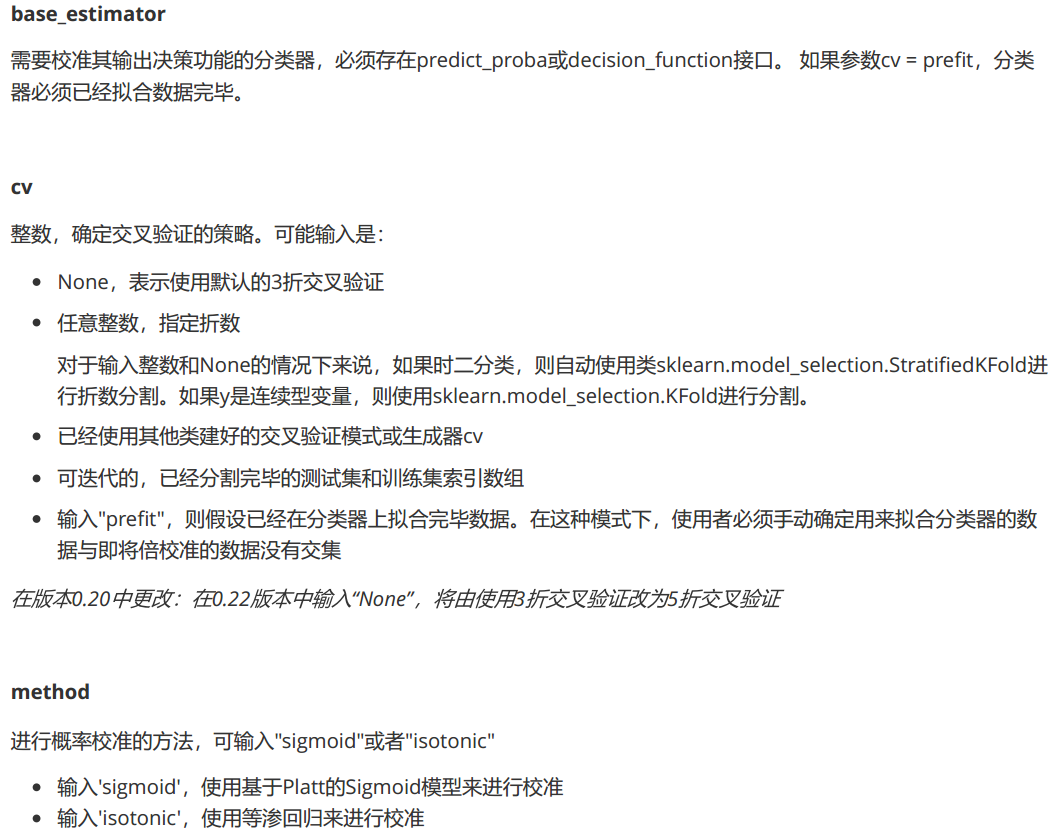1. 包装函数

def plot_calib(models, name, Xtrain, Xtest, Ytrain, Ytest, n_bins=10):fig, (ax1, ax2) = plt.subplots(1, 2, figsize=(20, 6))ax1.plot([0, 1], [0, 1], "k:", label="Perfectly calibrated")for clf, name_ in zip(models, name):clf.fit(Xtrain, Ytrain)y_pred = clf.predict(Xtest)# hasattr(obj,name)：查看一个类obj中是否存在名字为name的接口，存在则返回Trueif hasattr(clf, "predict_proba"):prob_pos = clf.predict_proba(Xtest)[:, 1]else:  # use decision functionprob_pos = clf.decision_function(Xtest)prob_pos = (prob_pos - prob_pos.min()) / (prob_pos.max() - prob_pos.min())#返回布里尔分数clf_score = brier_score_loss(Ytest, prob_pos, pos_label=1)trueproba, predproba = calibration_curve(Ytest, prob_pos, n_bins=n_bins)ax1.plot(predproba, trueproba, "s-", label="%s (%1.3f)" % (name_, clf_score))ax2.hist(prob_pos, range=(0, 1), bins=n_bins, label=name_, histtype="step", lw=2)ax2.set_ylabel("Distribution of probability")ax2.set_xlabel("Mean predicted probability")ax2.set_xlim([-0.05, 1.05])ax2.legend(loc=9)ax2.set_title("Distribution of probablity")ax1.set_ylabel("True probability for class 1")ax1.set_xlabel("Mean predcited probability")ax1.set_ylim([-0.05, 1.05])ax1.legend()ax1.set_title('Calibration plots(reliability curve)')plt.show()

1. 设实例化模型，设定模型的名字
from sklearn.calibration import CalibratedClassifierCV
from sklearn.naive_bayes import GaussianNB
from sklearn.linear_model import LogisticRegression
from sklearn.model_selection import train_test_split
from sklearn.datasets import make_classification as mc
import matplotlib.pyplot as plt
from sklearn.metrics import brier_score_loss
from sklearn.calibration import calibration_curveX, y = mc(n_samples=100000, n_features=20, n_classes=2, n_informative=2, n_redundant=10, random_state=42)
Xtrain, Xtest, Ytrain, Ytest = train_test_split(X, y, test_size=0.99, random_state=42)name = ["GaussianBayes", "Logistic", "Bayes+isotonic", "Bayes+sigmoid"]
gnb = GaussianNB()
models = [gnb,LogisticRegression(C=1., solver='lbfgs', max_iter=3000, multi_class='auto'),CalibratedClassifierCV(gnb, cv=2, method='isotonic'),CalibratedClassifierCV(gnb, cv=2, method='sigmoid')]

1. 基于函数进行绘图
plot_calib(models, name, Xtrain, Xtest, Ytrain, Ytest)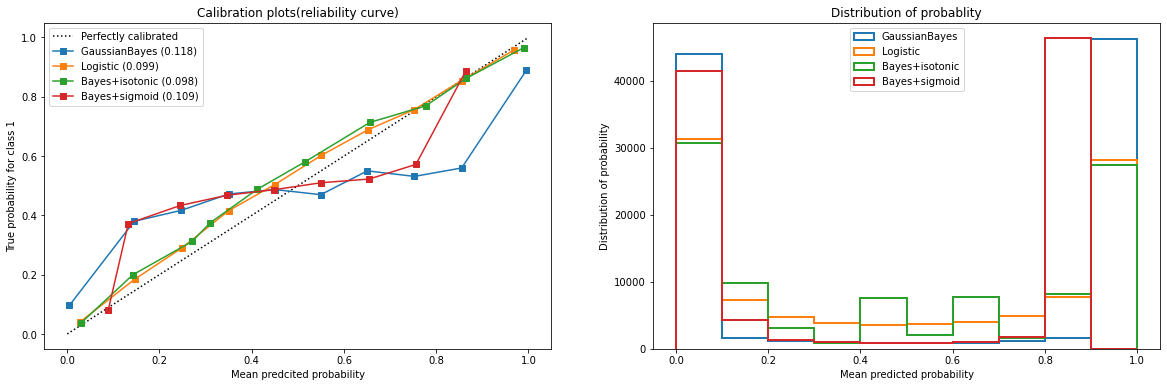1. 基于校准结果查看精确性的变化
gnb = GaussianNB().fit(Xtrain, Ytrain)
print("GaussianBayes:", gnb.score(Xtest, Ytest), brier_score_loss(Ytest, gnb.predict_proba(Xtest)[:, 1], pos_label=1))
gnb_isotonic = CalibratedClassifierCV(gnb, cv=2, method='isotonic').fit(Xtrain, Ytrain)
print("GaussianBayes+isotonic:", gnb_isotonic.score(Xtest, Ytest),brier_score_loss(Ytest, gnb_isotonic.predict_proba(Xtest)[:, 1], pos_label=1))
GaussianBayes: 0.8650606060606061 0.11760826355000836
GaussianBayes+isotonic: 0.8626767676767677 0.09833190251353853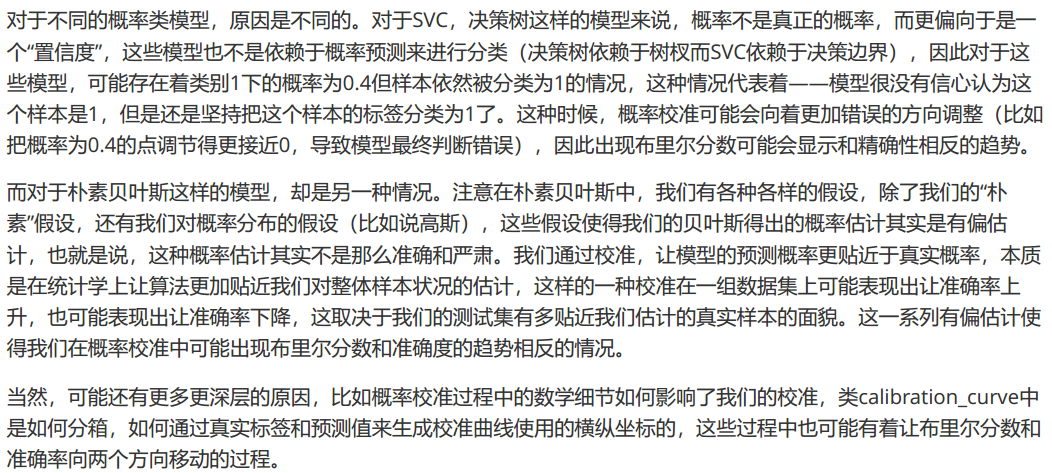1. 试试看对于SVC，哪种校准更有效呢？
from sklearn.svm import SVCname_svc = ["SVC", "Logistic", "SVC+isotonic", "SVC+sigmoid"]
svc = SVC(kernel="linear", gamma=1.0)
models_svc = [svc,LogisticRegression(C=1., solver='lbfgs', max_iter=3000, multi_class='auto'),CalibratedClassifierCV(svc, cv=2, method="isotonic"),CalibratedClassifierCV(svc, cv=2, method="sigmoid")]
plot_calib(models_svc, name_svc, Xtrain, Xtest, Ytrain, Ytest)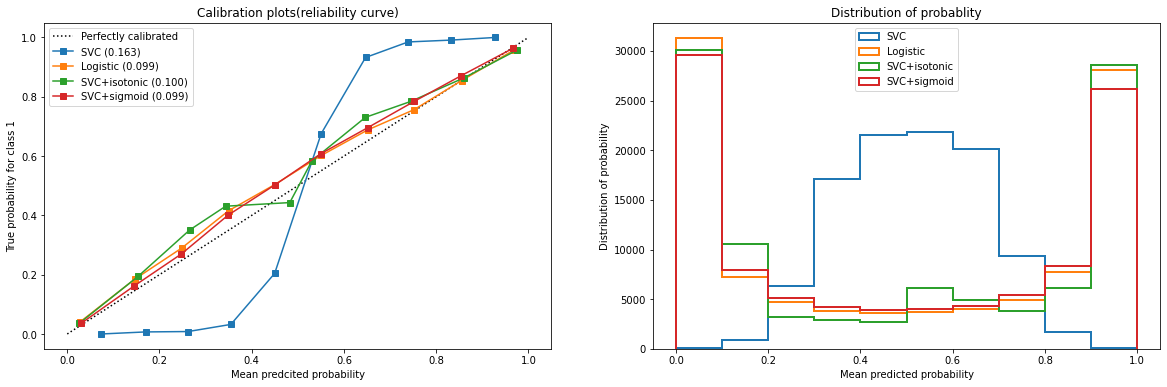name_svc = ["SVC", "SVC+isotonic", "SVC+sigmoid"]
svc = SVC(kernel="linear", gamma=1.0)
models_svc = [svc,CalibratedClassifierCV(svc, cv=2, method="isotonic"),CalibratedClassifierCV(svc, cv=2, method="sigmoid")]
for clf, name in zip(models_svc, name_svc):clf.fit(Xtrain, Ytrain)y_pred = clf.predict(Xtest)if hasattr(clf, "predict_proba"):prob_pos = clf.predict_proba(Xtest)[:, 1]else:prob_pos = clf.decision_function(Xtest)prob_pos = (prob_pos - prob_pos.min()) / (prob_pos.max() - prob_pos.min())clf_score = brier_score_loss(Ytest, prob_pos, pos_label=Ytest.max())score = clf.score(Xtest, Ytest)print("%s:" % name)print("\tBrier:%1.4f" % clf_score)print("\tAccuracy:%1.4f" % score)

SVC:Brier:0.1630Accuracy:0.8633
SVC+isotonic:Brier:0.0999Accuracy:0.8639
SVC+sigmoid:Brier:0.0987Accuracy:0.8634


## 2.3 多项式朴素贝叶斯以及其变化

### 2.3.1 多项式朴素贝叶斯MultinomialNB

• 算法简单且易于实现，计算效率高。
• 对于文本分类等高维离散特征的问题，多项式朴素贝叶斯算法通常表现良好。虽然sklearn中的多项式分布也可以处理连续型变量，但现实中，如果想要处理连续型变量，应当使用高斯朴素贝叶斯。
• 可以处理缺失特征数据。

• 特征之间的条件独立性假设可能不符合实际情况。
• 对于连续特征数据，多项式朴素贝叶斯算法不适用。
• 对于样本不平衡的数据集，可能会导致预测结果偏向于具有更多样本的类别。
• 多项式实验中的实验结果都很具体，它所涉及的特征往往是次数，频率，计数，出现与否这样的概念，这些概念都是离散的正整数，因此sklearn中的多项式朴素贝叶斯不接受负值的输入

sklearn.naive_bayes.MultinomialNB(alpha=1.0,fit_prior=True,class_prior=None)

• alpha：浮点型，可选参数(默认为1.0)，拉普拉斯或利德斯通平滑的参数，如果设置为0则表示完全没有平滑选项。但是需要注意的是，平滑相当于人为给概率加上一些噪音，因此设置得越大，多项式朴素贝叶斯的精确性会越低（虽然影响不是非常大），布里尔分数也会逐渐升高
• fit_prior：布尔值, 可不填(默认为True)。是否学习先验概率 P ( Y = c ) P(Y=c) 。如果设置为false，则不使用先验概率，而使用统一先验概率（uniform prior），即认为每个标签类出现的概率是 1 n _ c l a s s e s \frac{1}{n\_classes}
• class_prior：形似数组的结构，结构为(n_classes,)，可不填(默认为None)。类的先验概率 P ( Y = c ) P(Y=c) 。如果没有给出具体的先验概率则自动根据数据来进行计算。

1. 导入需要的模块和库
from sklearn.preprocessing import MinMaxScaler
from sklearn.naive_bayes import MultinomialNB
from sklearn.model_selection import train_test_split
from sklearn.datasets import make_blobs
from sklearn.metrics import brier_score_loss

1. 建立数据集
class_1 = 500
class_2 = 500
centers = [[0.0, 0.0], [2.0, 2.0]]  # 设定两个类别的中心
clusters_std = [0.5, 0.5]  # 设定两个类别的方差
X, y = make_blobs(n_samples=[class_1, class_2], centers=centers, cluster_std=clusters_std, random_state=0,shuffle=False)Xtrain, Xtest, Ytrain, Ytest = train_test_split(X, y, test_size=0.3, random_state=420)

1. 归一化，确保输入的矩阵不带有负数
# 先归一化，保证输入多项式朴素贝叶斯的特征矩阵中不带有负数
mms = MinMaxScaler().fit(Xtrain)
Xtrain_ = mms.transform(Xtrain)
Xtest_ = mms.transform(Xtest)

1. 建立多项式朴素贝叶斯分类器及查看相关属性
mnb = MultinomialNB().fit(Xtrain_, Ytrain)
# 重要属性class_log_prior_：返回每个标签类的对数先验概率log(P(Y))
# 由于概率永远是在[0,1]之间，因此对数先验概率返回的永远是负值
print(mnb.class_log_prior_)
# 可以使用np.exp(mnb.class_log_prior_)来查看真正的先验概率
print(np.exp(mnb.class_log_prior_))# 重要属性feature_log_prob_：返回一个固定标签类别下的每个特征的对数概率log(P(Xi|y))
print(mnb.feature_log_prob_)#重要属性class_count_：在fit时每个标签类别下包含的样本数。当fit接口中的sample_weight被设置时，该接口返回的值也会受到加权的影响
print(mnb.class_count_)

[-0.69029411 -0.69600841]
[0.50142857 0.49857143]
[[-0.76164788 -0.62903951][-0.72500918 -0.6622691 ]]
[351. 349.]

1. 分类器的效果如何呢？
mnb.predict(Xtest_)
mnb.predict_proba(Xtest_)  # 每个测试样本在每个标签类别上的概率
mnb.score(Xtest_, Ytest)  # 0.5433
brier_score_loss(Ytest, mnb.predict_proba(Xtest_)[:, 1], pos_label=1)  # 0.249778

0.24977828412546035

1. 效果不太理想，思考一下多项式贝叶斯的性质，我们能够做点什么呢？
# 来试试看把Xtrain转换成分类型数据
# 注意Xtrain是不用经过归一化的，因为做哑变量之后自然所有的数据不会有负数
from sklearn.preprocessing import KBinsDiscretizer  # 对连续型变量进行分箱kbs = KBinsDiscretizer(n_bins=10, encode='onehot').fit(Xtrain)  # 一个特征分10箱，进行哑变量处理
Xtrain_ = kbs.transform(Xtrain)  # Xtrain_:(700,20)
Xtest_ = kbs.transform(Xtest)mnb = MultinomialNB().fit(Xtrain_, Ytrain)
print(mnb.score(Xtest_, Ytest), brier_score_loss(Ytest, mnb.predict_proba(Xtest_)[:, 1], pos_label=1))

0.9966666666666667 0.0014593932778211862


### 2.3.2 伯努利朴素贝叶斯BernoulliNB

classsklearn.naive_bayes.BernoulliNB(alpha=1.0,binarize=0.0,fit_prior=True,class_prior=None)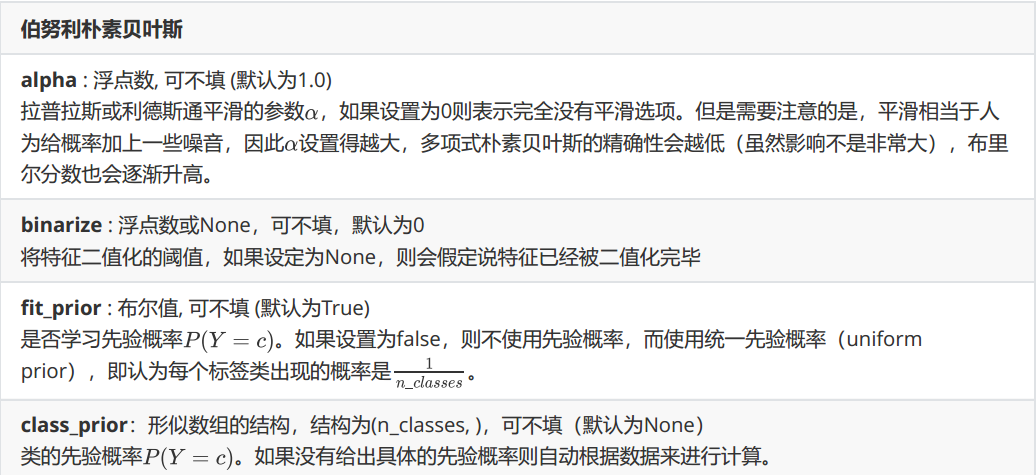from sklearn.naive_bayes import BernoulliNB# 普通来说我们应该使用二值化的类sklearn.preprocessing.Binarizer来将特征一个个二值化
# 然而这样效率过低，因此我们选择归一化之后直接设置一个阈值
mms = MinMaxScaler().fit(Xtrain)  # 归一化
Xtrain_ = mms.transform(Xtrain)
Xtest_ = mms.transform(Xtest)# 不设置二值化
bnl_ = BernoulliNB().fit(Xtrain_, Ytrain)
print(bnl_.score(Xtest_, Ytest), brier_score_loss(Ytest, bnl_.predict_proba(Xtest_)[:, 1], pos_label=1))# 设置二值化阈值为0.5
bnl = BernoulliNB(binarize=0.5).fit(Xtrain_, Ytrain)
print(bnl.score(Xtest_, Ytest), brier_score_loss(Ytest, bnl.predict_proba(Xtest_)[:, 1], pos_label=1))

0.49666666666666665 0.25000009482193225
0.9833333333333333 0.010405875827339534


### 2.3.3 探索贝叶斯：样本不均衡问题

1. 导入需要的模块，建立样本不平衡的数据集
from sklearn.naive_bayes import GaussianNB, MultinomialNB, BernoulliNB
from sklearn.preprocessing import KBinsDiscretizer
from sklearn.datasets import make_blobs
from sklearn.metrics import brier_score_loss as BS, recall_score, roc_auc_score as AUC
from sklearn.model_selection import train_test_split
import numpy as npclass_1 = 50000  # 多数类别1有50000个样本
class_2 = 500  # 少数类别2只有500个
centers = [[0.0, 0.0], [5.0, 5.0]]  # 设定两个类别的中心
clusters_std = [3, 1]  # 设定两个类别的方差
X, y = make_blobs(n_samples=[class_1, class_2], centers=centers, cluster_std=clusters_std, random_state=0,shuffle=False)

1. 查看所有贝叶斯在样本不平衡数据集上的表现
name = ['MultinomialNB', 'GaussianNB', 'BernoulliNB']
models = [MultinomialNB(), GaussianNB(), BernoulliNB()]for name, clf in zip(name, models):Xtrain, Xtest, Ytrain, Ytest = train_test_split(X, y, test_size=0.3, random_state=420)if name != "GaussianNB":kbs = KBinsDiscretizer(n_bins=10, encode='onehot').fit(Xtrain)Xtrain = kbs.transform(Xtrain)Xtest = kbs.transform(Xtest)clf = clf.fit(Xtrain, Ytrain)y_pred = clf.predict(Xtest)proba = clf.predict_proba(Xtest)[:, 1]  # 获取预测为1的概率score = clf.score(Xtest, Ytest)print(name)print("\tBrier:{:.3f}".format(BS(Ytest, proba, pos_label=1)))print("\tAccuracy:{:.3f}".format(score))print("\tRecall:{:.3f}".format(recall_score(Ytest, y_pred)))print("\tAUC:{:.3f}".format(AUC(Ytest, proba)))

MultinomialNBBrier:0.007Accuracy:0.990Recall:0.000AUC:0.991
GaussianNBBrier:0.006Accuracy:0.990Recall:0.438AUC:0.993
BernoulliNBBrier:0.009Accuracy:0.987Recall:0.771AUC:0.987


### 2.3.4 改进多项式朴素贝叶斯：补集朴素贝叶斯ComplementNB

• Rennie, J. D., Shih, L., Teevan, J., & Karger, D. R. (2003). Tackling the poor assumptions of naive bayes text classifiers. In ICML (Vol. 3, pp. 616-623).

sklearn.naive_bayes.ComplementNB(alpha=1.0,fit_prior=True,class_prior=None,norm=False)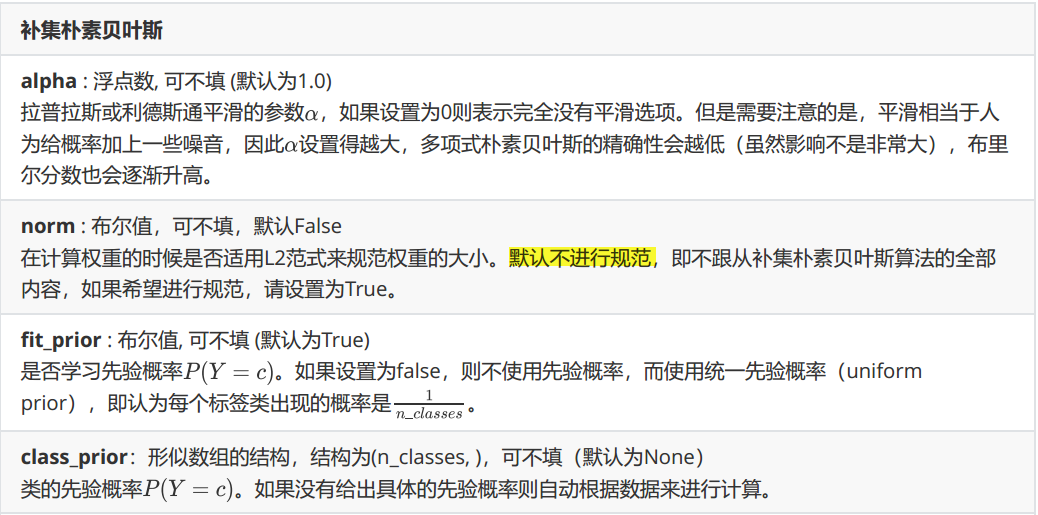from sklearn.naive_bayes import ComplementNB
from time import time
import datetimename = ["Multinomial", "Gaussian", "Bernoulli", "Complement"]
models = [MultinomialNB(), GaussianNB(), BernoulliNB(), ComplementNB()]for name, clf in zip(name, models):start = time()Xtrain, Xtest, Ytrain, Ytest = train_test_split(X, y, test_size=0.3, random_state=420)if name != "Gaussian":kbs = KBinsDiscretizer(n_bins=10, encode='onehot').fit(Xtrain)Xtrain = kbs.transform(Xtrain)Xtest = kbs.transform(Xtest)clf = clf.fit(Xtrain, Ytrain)y_pred = clf.predict(Xtest)proba = clf.predict_proba(Xtest)[:, 1]score = clf.score(Xtest, Ytest)print(name)print("\tBrier:{:.3f}".format(BS(Ytest, proba, pos_label=1)))print("\tAccuracy:{:.3f}".format(score))print("\tRecall:{:.3f}".format(recall_score(Ytest, y_pred)))print("\tAUC:{:.3f}".format(AUC(Ytest, proba)))print(datetime.datetime.fromtimestamp(time() - start).strftime("%M:%S:%f"))

MultinomialBrier:0.007Accuracy:0.990Recall:0.000AUC:0.991
00:00:031265
GaussianBrier:0.006Accuracy:0.990Recall:0.438AUC:0.993
00:00:031245
BernoulliBrier:0.009Accuracy:0.987Recall:0.771AUC:0.987
00:00:036370
ComplementBrier:0.038Accuracy:0.953Recall:0.987AUC:0.991
00:00:031252


# 3 案例：贝叶斯分类器做文本分类

1. 数据准备： 收集并准备用于训练和测试的文本数据集。数据集应包括带有标签的文本样本，每个样本都与一个或多个类别相关联。

2. 特征提取： 将文本数据转换为可供机器学习算法处理的特征表示。常用的特征提取方法包括词袋模型（Bag of Words）、TF-IDF、Word2Vec等。

3. 训练模型： 使用标记好的训练数据来训练文本分类模型。常见的分类算法包括朴素贝叶斯、支持向量机（SVM）、决策树、深度学习模型如卷积神经网络（CNN）和循环神经网络（RNN）等。

4. 模型评估： 使用测试数据评估训练得到的模型的性能。常用的评估指标包括准确率、精确率、召回率、F1分数等。

5. 模型应用： 使用训练好的模型对新的未知文本进行分类预测。

## 3.1 文本编码技术简介

### 3.1.1 单词计数向量

from sklearn.feature_extraction.text import CountVectorizer
import pandas as pdsample = ["Machine learning is fascinating, it is wonderful","Machine learning is a sensational technology","Elsa is a popular character"]vec = CountVectorizer()
X = vec.fit_transform(sample)# 使用接口get_feature_names()调用每个列的名称
# 注意稀疏矩阵是无法输入pandas的
CVresult = pd.DataFrame(X.toarray(), columns=vec.get_feature_names_out())
CVresult

characterelsafascinatingisitlearningmachinepopularsensationaltechnologywonderful
000121110001
100010110110
211010001000

1. 简单直观： 单词计数向量的生成过程相对简单直观，只需统计每个文档中每个单词出现的次数即可。
2. 保留全局信息： 单词计数向量保留了每个单词在整个文本集合中的全局出现频率，可以反映单词的重要性和稀有性。
3. 适用性广泛： 单词计数向量适用于大多数文本分类任务，特别是在较小的数据集上表现良好。
4. 高效性： 生成单词计数向量的过程可以高度并行化，适合在大规模文本数据上进行处理。

1. 忽略单词顺序： 单词计数向量忽略了单词在文本中的顺序信息，将文本表示为无序的集合。这可能丢失了一些重要的语义和上下文信息。
2. 受词频影响： 单词计数向量容易受到常用词汇的影响，例如常见的停用词(is 、the)，这些词在大多数文本中出现频率很高，但对文本分类任务的贡献较小
3. 向量维度大： 单词计数向量的维度通常较大，取决于词汇表的大小。这可能导致高维稀疏向量的表示，增加了存储和计算的开销。
4. 无法处理新词： 单词计数向量在训练阶段构建词汇表，但对于未在训练数据中出现的新词，无法准确地进行编码。

### 3.1.2 TF-IDF

TF-IDF（Term Frequency-Inverse Document Frequency）是一种常用的文本特征表示方法，用于衡量一个词对于一个文档集合中的某个文档的重要程度。

TF-IDF的计算基于两个概念：词频（Term Frequency）和逆文档频率（Inverse Document Frequency）。

1. 词频（Term Frequency，TF）： 衡量一个词在文档中的出现频率。词频可以通过简单地计算某个词在文档中出现的次数来表示，或者进行归一化处理，例如使用相对词频（频率除以文档中的总词数）或对数词频（取对数后缩小频率范围）。

2. 逆文档频率（Inverse Document Frequency，IDF）： 衡量一个词的普遍重要程度。逆文档频率通过计算文档集合中包含某个词的文档数目的倒数来表示。常见的计算方式是取总文档数除以包含该词的文档数的对数。

TF-IDF的计算公式为：TF-IDF = TF * IDF

TF-IDF的优点包括：

• 能够提取出在特定文档中频繁出现且在整个文档集合中较为罕见的关键词。
• 能够突出词语在文档中的重要性，从而更好地表示文档的特征。

TF-IDF的应用广泛，特别适用于文本分类、信息检索和文本相似度计算等任务。它能够帮助识别关键词、过滤停用词，并提供重要性排序的特征表示。

IDF的大小与一个词的常见程度成反比，这个词越常见，编码后为它设置的权重会倾向于越小，以此来压制频繁出现的一些无意义的词

from sklearn.feature_extraction.text import TfidfVectorizer as TFIDFvec = TFIDF()
X = vec.fit_transform(sample)
TFIDFresult = pd.DataFrame(X.toarray(), columns=vec.get_feature_names_out())
TFIDFresult

characterelsafascinatingisitlearningmachinepopularsensationaltechnologywonderful
00.0000000.0000000.4243960.5013100.4243960.3227640.3227640.0000000.0000000.0000000.424396
10.0000000.0000000.0000000.3154440.0000000.4061920.4061920.0000000.5340930.5340930.000000
20.5464540.5464540.0000000.3227450.0000000.0000000.0000000.5464540.0000000.0000000.000000
# 使用TF-IDF编码之后，出现得多的单词的权重theta被降低了么？
print(CVresult.sum(axis=0) / CVresult.sum(axis=0).sum())
print('-' * 50)
print(TFIDFresult.sum(axis=0) / TFIDFresult.sum(axis=0).sum())
# 将原本出现次数比较多的词压缩我们的权重
# 将原本出现次数比较少的词增加我们的权重

character      0.0625
elsa           0.0625
fascinating    0.0625
is             0.2500
it             0.0625
learning       0.1250
machine        0.1250
popular        0.0625
sensational    0.0625
technology     0.0625
wonderful      0.0625
dtype: float64
--------------------------------------------------
character      0.083071
elsa           0.083071
fascinating    0.064516
is             0.173225
it             0.064516
learning       0.110815
machine        0.110815
popular        0.083071
sensational    0.081192
technology     0.081192
wonderful      0.064516
dtype: float64


## 3.2 探索文本数据

from sklearn.datasets import fetch_20newsgroupsdata = fetch_20newsgroups()# 通常我们使用data来查看data里面到底包含了什么内容，但由于fetch_20newsgourps这个类加载出的数据巨大，数据结构中混杂很多文字，因此很难去看清# 不同类型的新闻
print(data.target_names)# 其实fetch_20newsgroups也是一个类，既然是类，应该就有可以调用的参数
# 面对简单数据集，往往在实例化的过程中什么都不写，但是现在data中数据量太多，不方便探索
# 因此我们需要来看看我们的类fetch_20newsgroups都有什么样的参数可以帮助我们

['alt.atheism', 'comp.graphics', 'comp.os.ms-windows.misc', 'comp.sys.ibm.pc.hardware', 'comp.sys.mac.hardware', 'comp.windows.x', 'misc.forsale', 'rec.autos', 'rec.motorcycles', 'rec.sport.baseball', 'rec.sport.hockey', 'sci.crypt', 'sci.electronics', 'sci.med', 'sci.space', 'soc.religion.christian', 'talk.politics.guns', 'talk.politics.mideast', 'talk.politics.misc', 'talk.religion.misc']


sklearn.datasets.fetch_20newsgroups(data_home=None,subset=’train’,categories=None, shuffle=True, random_state=42, remove=(), download_if_missing=True)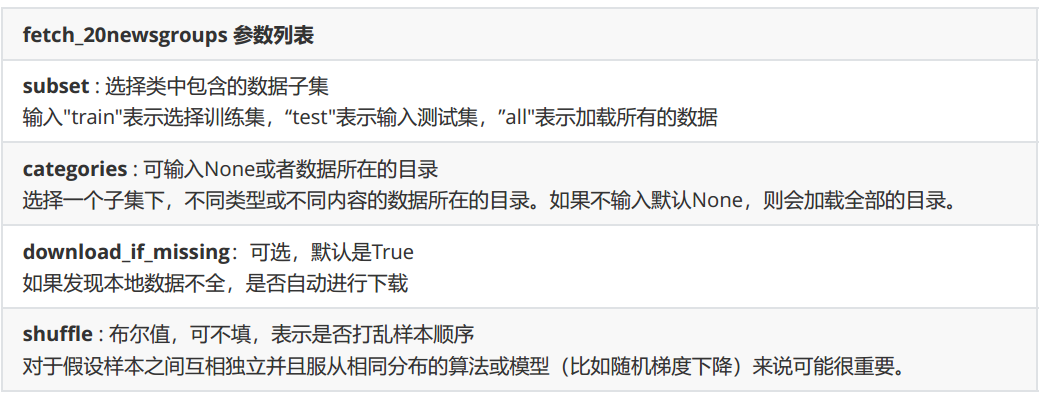import numpy as np
import pandas as pd# 提取四种类型文章的数据集：即这是一个四分类任务
catagories = ["sci.space", "rec.sport.hockey", "talk.politics.guns", "talk.politics.mideast"]
train = fetch_20newsgroups(subset='train', categories=catagories)
test = fetch_20newsgroups(subset='test', categories=catagories)# train 里面依然是类字典结构，我们可以通过使用键的方式来提取内容
print(train.target_names)# 查看总共有多少篇文章
print(len(train.data))# 查看第一篇文章的内容
# print(train.data)# 查看标签
print(train.target, len(train.target))# 是否存在样本不均衡问题
for i in range(4):print(i, (train.target == i).sum() / len(train.target))# 可以看出没有样本不均衡问题

['rec.sport.hockey', 'sci.space', 'talk.politics.guns', 'talk.politics.mideast']
2303
[0 1 3 ... 1 0 1] 2303
0 0.26052974381241856
1 0.25749023013460703
2 0.23708206686930092
3 0.24489795918367346


## 3.3 使用TF-IDF将文本数据编码

from sklearn.feature_extraction.text import TfidfVectorizer as TFIDFXtrain = train.data
Xtest = test.data
Ytrain = train.target
Ytest = test.targettfidf = TFIDF().fit(Xtrain)
Xtrain_ = tfidf.transform(Xtrain)
Xtest_ = tfidf.transform(Xtest)tosee = pd.DataFrame(Xtrain_.toarray(), columns=tfidf.get_feature_names_out())
# tosee.shape (2303, 40725)

00000000000000000000000021000062david42000152000246000256...zwrmzxzx6wrezxpzxqizyzygzzzz_g9q3zzzzzz
00.00.0000000.00.00.00.00.00.00.00.0...0.00.00.00.00.00.00.00.00.00.0
10.00.0000000.00.00.00.00.00.00.00.0...0.00.00.00.00.00.00.00.00.00.0
20.00.0580460.00.00.00.00.00.00.00.0...0.00.00.00.00.00.00.00.00.00.0
30.00.0000000.00.00.00.00.00.00.00.0...0.00.00.00.00.00.00.00.00.00.0
40.00.0000000.00.00.00.00.00.00.00.0...0.00.00.00.00.00.00.00.00.00.0

5 rows × 40725 columns

## 3.4 在贝叶斯上分别建模，查看结果

from sklearn.naive_bayes import MultinomialNB, BernoulliNB, ComplementNB
from sklearn.metrics import log_lossname = ['MultinomialNB', 'ComplementNB', 'BernoulliNB']# 注意高斯贝叶斯不接受稀疏矩阵，故这里不用
models = [MultinomialNB(), ComplementNB(), BernoulliNB()]for name, clf in zip(name, models):clf.fit(Xtrain_, Ytrain)y_pred = clf.predict_proba(Xtest_)proba = clf.predict_proba(Xtest_)log_loss_score = log_loss(Ytest, proba)score = clf.score(Xtest_, Ytest)print(name)print("\tlog_loss:{:.3f}".format(log_loss_score))print("\tAccuracy:{:.3f}".format(score))

MultinomialNBlog_loss:0.268Accuracy:0.975
ComplementNBlog_loss:0.331Accuracy:0.986
BernoulliNBlog_loss:2.083Accuracy:0.902


from sklearn.calibration import CalibratedClassifierCVname = ["Multinomial", "Multinomial + Isotonic", "Multinomial + Sigmoid", "Complement", "Complement + Isotonic", "Complement + Sigmoid", "Bernoulli", "Bernoulli + Isotonic", "Bernoulli + Sigmoid"]models = [MultinomialNB(), CalibratedClassifierCV(MultinomialNB(), cv=2, method='isotonic'), CalibratedClassifierCV(MultinomialNB(), cv=2, method='sigmoid'), ComplementNB(), CalibratedClassifierCV(ComplementNB(), cv=2, method='isotonic'), CalibratedClassifierCV(ComplementNB(), cv=2, method='sigmoid'), BernoulliNB(), CalibratedClassifierCV(BernoulliNB(), cv=2, method='isotonic'), CalibratedClassifierCV(BernoulliNB(), cv=2, method='sigmoid')]
for name, clf in zip(name, models):clf.fit(Xtrain_, Ytrain)y_pred = clf.predict_proba(Xtest_)proba = clf.predict_proba(Xtest_)log_loss_score = log_loss(Ytest, proba)score = clf.score(Xtest_, Ytest)print(name)print("\tlog_loss:{:.3f}".format(log_loss_score))print("\tAccuracy:{:.3f}".format(score))

Multinomiallog_loss:0.268Accuracy:0.975
Multinomial + Isotoniclog_loss:0.140Accuracy:0.973
Multinomial + Sigmoidlog_loss:0.091Accuracy:0.973
Complementlog_loss:0.331Accuracy:0.986
Complement + Isotoniclog_loss:0.071Accuracy:0.985
Complement + Sigmoidlog_loss:0.071Accuracy:0.986
Bernoullilog_loss:2.083Accuracy:0.902
Bernoulli + Isotoniclog_loss:0.229Accuracy:0.937
Bernoulli + Sigmoidlog_loss:0.443Accuracy:0.879


### sqlalchemy从入门到熟悉（一）

sqlalchemy从入门到熟悉&#xff08;一&#xff09; 简介 SQLAlchemy SQL工具包和对象关系映射器是一套用于处理数据库和Python的综合工具。它有几个不同的功能领域&#xff0c;可以单独使用或组合使用。SQLAlchemy的两个最重要的面向前端的部分是对象关系映射器&#xff08;O…

### Python代码写好了怎么运行

Python代码写好了怎么运行&#xff1f;相信问这样问题的朋友一定是刚刚入门Python的初学者。本文就来为大家详细讲讲如何运行Python代码。 一般来讲&#xff0c;运行Python代码的方式有两种&#xff0c;一是在Python交互式命令行下运行&#xff1b;另一种是使用文本编辑器&…

### logstash 采集的文件mv后

1.logstash [oswatch@rce1 conf]$cat test.conf input { file { path=>["/tmp/test/test.log*"] } } output { stdout { codec=>rubydebug{} } } 2.python脚本: [oswatch@rce1 conf]$ cat t1.py #!/usr/bin/python # -*- coding: UTF-…

### virtualbox报错

virtualbox 报错 See “systemctl status virtualbox.service” and “journalctl -xe” for details. invoke-rc.d: initscript virtualbox, action “restart” failed. ● virtualbox.service - LSB: VirtualBox Linux kernel module Loaded: loaded (/etc/init.d/virtualbo…

### 【js】对象属性的拦截和Proxy代理与Reflect映射的用法与区别

✍️ 作者简介: 前端新手学习中。 &#x1f482; 作者主页: 作者主页查看更多前端教学 &#x1f393; 专栏分享&#xff1a;css重难点教学 Node.js教学 从头开始学习 ajax学习 文章目录 对象属性的拦截介绍SetGet 对象的拦截介绍使用对象属性拦截和对象拦截区别练习题 映射…